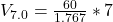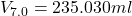Question

A 72.0 g sample of an organic solid is dissolved in 180mL of water. The solid is extracted using one 60 mL extraction in the first extraction of an organic solvent which has a partition (distribution) coefficient with water of 10. The first extraction removed 55.4 g of solid from water. What are the numbers that need to go in box A and B to calculate the volume of solvent (y) that would be necessary to remove an additional 7.0g from the remaining sample dissolved in water. You DON’T have to complete the calculation to solve for y.

1.Tryphena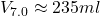Explanation:

From the question we are told that

mass of sample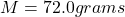volume of water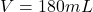volume for extraction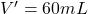partition (distribution) coefficient water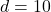initial extraction removal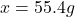Generally the equation for the weight of sample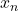is mathematically given by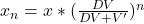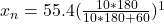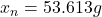Generally the weight extracted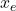is therefore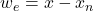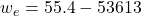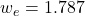is extracted with 60ml solvent .

Therefore volume of solvent (y) that would be necessary to remove an additional 7.0g